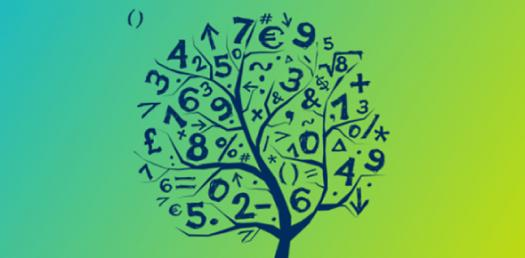# Primary Maths

9 Questions | Attempts: 3140
ShareSettingsThis is a general mathematics quiz for upper primary school students.

• 1.
A 2D shape with 4 sides and 4 angles could be called
• A.

• B.

A Square

• C.

A Rectangle

• D.

Any of the above

• 2.
The word Millimetre literally means
• A.

One tenth of a centimetre

• B.

One thousandth of a metre

• C.

One millionth of a metre

• D.

A REALLY long millipede

• 3.
12 X _ = 72
• 4.
½ X 10 =
• A.

20

• B.

5

• C.

1

• D.

D

• 5.
0.60 is the same as
• A.

60/10

• B.

6/10

• C.

6/100

• D.

6/1

• 6.
A triangle is
• A.

Anything pointy

• B.

A sandwich

• C.

Any shape with three sides and three angles

• D.

The roof of a house

• 7.
The 3 dimensions in ‘3D’ are
• A.

Up/down, left/right and in/out

• B.

Space, time and matter

• C.

Darlek, Human and Aliens

• D.

A really cool Sci-fi series.

• 8.
52 =
• A.

10

• B.

7

• C.

5

• D.

25

• 9.
What is the next number in this pattern256, 128, 64, 32, __
• A.

16

• B.

8

• C.

31

• D.

0

## Related TopicsBack to top
×

Wait!
Here's an interesting quiz for you.